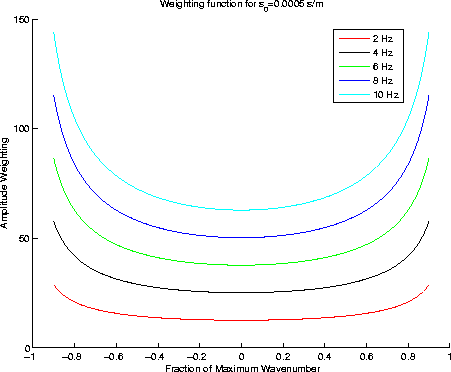Next: Waveform Inversion in Riemannian Up: Shragge: TWVA Previous: Conclusion

## REFERENCES

Operto, S., J. Virieux, et al., 2006, 3D frequency-domain finite-difference modeling of acoustic waves using a massively parallel direct solver: A feasibility study: 75th SEG Ann. Gen. Meeting Conference and Exhibition, Expanded Abstracts.

Pratt, R. and M. Worthington, 1989, The application of diffraction tomography to cross-hole seismic data: Geophysics, 53, 1284-1294.

Sava, P. and B. Biondi, 2004, Wave-equation migration velocity analysis. I. Theory: Geophysical Prospecting, 52, 593-606.

Sava, P. and S. Fomel, 2005, Riemannian wavefield extrapolation: Geophysics, 70, T45-T56.

Shragge, J., 2006, Non-orthogonal Riemannian wavefield extrapolation: 75th SEG Ann. Gen. Meeting and Exhibition, Expanded Abstracts, 2236-2240.

Sirgue, L. and R. Pratt, 2004, Efficient waveform inversion and imaging: A strategy for selecting temporal frequencies: Geophysics, 69, 231-248.

Stekl, I. and R. Pratt, 1998, Accurate visco-elastic modeling frequency-domain finite differences using rotated operators: Geophysics, 63, 1779-1794.

Woodward, M., 1992, Wave-equation tomography: Geophysics, 57, 15-26.

## APPENDIX A

This appendix develops a WEMVA scattering operator Sava and Biondi (2004) for use in transmission wavefield waveform inversion. The extrapolation operator,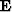, is given by,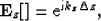(29)
where kz is the depth wavenumber andis the extrapolation depth step. The extrapolation wavenumber in depth is given by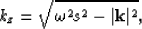(30)
where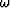is temporal frequency and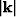is the horizontal wavenumber magnitude.

The vertical wavenumber can be separated into two components, one corresponding to the background medium,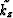, and one corresponding to a perturbation,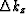, such that,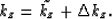(31)
In a first-order approximation, we can relate these two extrapolation wavenumbers by a Taylor-series expansion,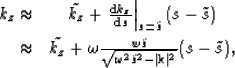(32)
where s(x,z) is the slowness andcorresponds to a background slowness.

Within any depth slab we can extrapolate the wavefield from the top, either in the perturbed or in the background medium. The wavefields at the bottom of the slab,and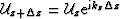, related by,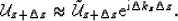(33)
Equation 34 is a direct statement of the Rytov approximation, because the wavefields at the bottom of the slab correspond to different phase shifts related by a linear equation. Thus, we obtain the wavefield perturbationat the bottom of the slab by subtracting the background wavefield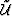from the perturbed wavefield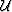: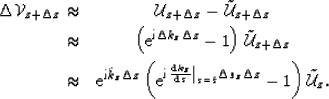(34)

For the Born approximation, we further assume that the wavefield differences are small so that we linearize the exponential function according to. With this approximation we write the following downward continued scattered wavefield,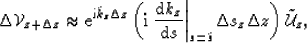(35)
which, in operator form is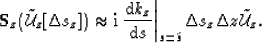(36)

The Born operator may be implemented in the Fourier domain relative to a constant reference slowness in any individual slab. In this case,(37)
whereis a damping parameter to avoid division by zero.

Figureshows the amplitude weighting demanded by the filter in equation 38 for five different frequencies for slowness 0.5 s/km.

 KXfilterFigure 1 Example of the Born amplitude weighting function demanded by the WEMVA theory for a slowness of 0.5 s/km and a damping factor of 0.001.